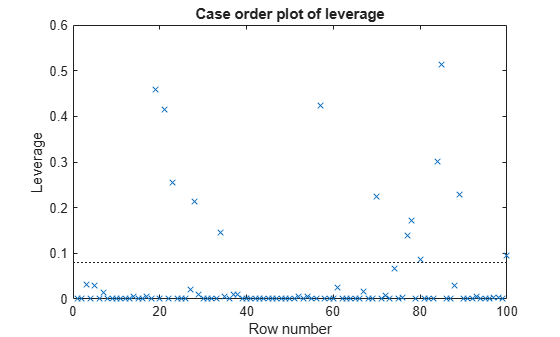# Generalized Linear Model Workflow

This example shows how to fit a generalized linear model and analyze the results. A typical workflow involves these steps: import data, fit a generalized linear model, test its quality, modify the model to improve its quality, and make predictions based on the model. In this example, you use the Fisher iris data to compute the probability that a flower is in one of two classes.

`load fisheriris`

Extract rows 51 to 150, which have the classification versicolor or virginica.

`X = meas(51:end,:);`

Create logical response variables that are `true` for versicolor and `false` for virginica.

`y = strcmp('versicolor',species(51:end));`

### Fit Generalized Linear Model

Fit a binomial generalized linear model to the data.

`mdl = fitglm(X,y,'linear','Distribution','binomial')`
```mdl = Generalized linear regression model: logit(y) ~ 1 + x1 + x2 + x3 + x4 Distribution = Binomial Estimated Coefficients: Estimate SE tStat pValue ________ ______ _______ ________ (Intercept) 42.638 25.708 1.6586 0.097204 x1 2.4652 2.3943 1.0296 0.30319 x2 6.6809 4.4796 1.4914 0.13585 x3 -9.4294 4.7372 -1.9905 0.046537 x4 -18.286 9.7426 -1.8769 0.060529 100 observations, 95 error degrees of freedom Dispersion: 1 Chi^2-statistic vs. constant model: 127, p-value = 1.95e-26 ```

According to the model display, some p-values in the `pValue` column are not small, which implies that you can simplify the model.

### Examine and Improve Model

Determine if 95% confidence intervals for the coefficients include 0. If so, you can remove the model terms with those intervals.

`confint = coefCI(mdl)`
```confint = 5×2 -8.3984 93.6740 -2.2881 7.2185 -2.2122 15.5739 -18.8339 -0.0248 -37.6277 1.0554 ```

Only the fourth predictor `x3` has a coefficient whose confidence interval does not include 0.

The coefficients of `x1` and `x2` have large p-values and their 95% confidence intervals include 0. Test whether both coefficients can be zero. Specify a hypothesis matrix to select the coefficients of `x1` and `x2`.

```M = [0 1 0 0 0 0 0 1 0 0]; p = coefTest(mdl,M)```
```p = 0.1442 ```

The p-value is approximately 0.14, which is not small. Remove `x1` and `x2` from the model.

`mdl1 = removeTerms(mdl,'x1 + x2')`
```mdl1 = Generalized linear regression model: logit(y) ~ 1 + x3 + x4 Distribution = Binomial Estimated Coefficients: Estimate SE tStat pValue ________ ______ _______ __________ (Intercept) 45.272 13.612 3.326 0.00088103 x3 -5.7545 2.3059 -2.4956 0.012576 x4 -10.447 3.7557 -2.7816 0.0054092 100 observations, 97 error degrees of freedom Dispersion: 1 Chi^2-statistic vs. constant model: 118, p-value = 2.3e-26 ```

Alternatively, you can identify important predictors using `stepwiseglm`.

`mdl2 = stepwiseglm(X,y,'constant','Distribution','binomial','Upper','linear')`
```1. Adding x4, Deviance = 33.4208, Chi2Stat = 105.2086, PValue = 1.099298e-24 2. Adding x3, Deviance = 20.5635, Chi2Stat = 12.8573, PValue = 0.000336166 3. Adding x2, Deviance = 13.2658, Chi2Stat = 7.29767, PValue = 0.00690441 ```
```mdl2 = Generalized linear regression model: logit(y) ~ 1 + x2 + x3 + x4 Distribution = Binomial Estimated Coefficients: Estimate SE tStat pValue ________ ______ _______ ________ (Intercept) 50.527 23.995 2.1057 0.035227 x2 8.3761 4.7612 1.7592 0.078536 x3 -7.8745 3.8407 -2.0503 0.040334 x4 -21.43 10.707 -2.0014 0.04535 100 observations, 96 error degrees of freedom Dispersion: 1 Chi^2-statistic vs. constant model: 125, p-value = 5.4e-27 ```

The p-value (`pValue`) for `x2` in the coefficient table is greater than 0.05, but `stepwiseglm` includes `x2` in the model because the p-value (`PValue`) for adding `x2` is smaller than 0.05. The `stepwiseglm` function computes `PValue` using the fits with and without `x2`, whereas the function computes `pValue` based on an approximate standard error computed only from the final model. Therefore, `PValue` is more reliable than `pValue`.

### Identify Outliers

Examine a leverage plot to look for influential outliers.

`plotDiagnostics(mdl2,'leverage')`An observation can be considered an outlier if its leverage substantially exceeds `p/n`, where `p` is the number of coefficients and `n` is the number of observations. The dotted reference line is a recommended threshold, computed by `2*p/n`, which corresponds to 0.08 in this plot. Some observations have leverage values larger than `10*p/n` (that is, 0.40). Identify these observation points.

`idxOutliers = find(mdl2.Diagnostics.Leverage > 10*mdl2.NumCoefficients/mdl2.NumObservations)`
```idxOutliers = 4×1 19 21 57 85 ```

See if the model coefficients change when you fit a model excluding these points.

```oldCoeffs = mdl2.Coefficients.Estimate; mdl3 = fitglm(X,y,'linear','Distribution','binomial', ... 'PredictorVars',2:4,'Exclude',idxOutliers); newCoeffs = mdl3.Coefficients.Estimate; disp([oldCoeffs newCoeffs])```
``` 50.5268 44.0085 8.3761 5.6361 -7.8745 -6.1145 -21.4296 -18.1236 ```

The model coefficients in `mdl3` are different from those in mdl2. This result implies that the responses at the high-leverage points are not consistent with the predicted values from the reduced model.

### Predict Probability of Being Versicolor

Use `mdl3` to predict the probability that a flower with average measurements is versicolor. Generate confidence intervals for the prediction.

`[newf,newc] = predict(mdl3,mean(X))`
```newf = 0.4558 ```
```newc = 1×2 0.1234 0.8329 ```

The model gives almost a 46% probability that the average flower is versicolor, with a wide confidence interval.